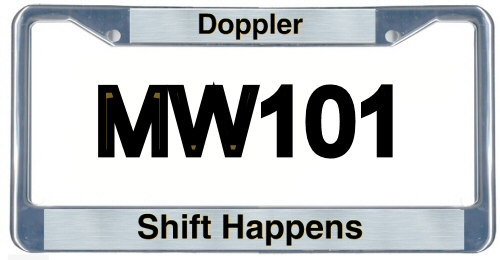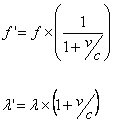# Doppler Shift

New for September 2008!What kind of a nerd would put that plate on his automobile? ...don't answer that!

Doppler shift happens when a signal either originates or is bounced off of a "target" that is moving with respect to the observer (the radar). The Doppler shift is what allows a radar to determine the velocity of the target.

The equation below shows the relationship between the observed frequency and wavelength compared to the "original" frequency and wavelength. Velocity is the component radial to the observer (as opposed to orthogonal), and is positive by convention if the object is moving away from the observer. Thus the frequency is shifted higher if the object is approaching, and lower if it is receding (think about the sound that an ambulance makes when it drives toward you, then away from you).This equation can be applied to sound waves as well as electromagnetic waves. In radar, we are talking about EM waves, so the velocity of the object is compared to the velocity of light. Notice that the speed of light can be easily 10,000,000,000 times faster than the object. It is quite remarkable that a radar can perform the comparison of the incident and returned frequencies to such a degree that you can resolve such a slight difference. The most important thing is to use a stable source, meaning that its frequency does not change much at all over time. Then a simple mixer arrangement can be used to determine the Doppler frequency.

Author : Unknown Editor